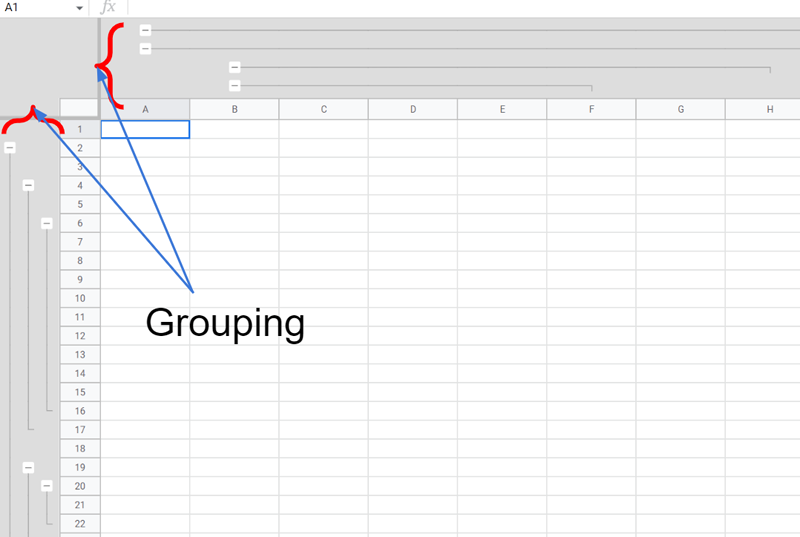GistsIn this post, I would like to introduce 2 sample scripts for copying and deleting the dimension groups in Google Spreadsheet using Google Apps Script.

Unfortunately, in the current stage, all dimension groups cannot be copied by one action, and also, all dimension groups cannot be deleted by one action. In this post, these can be achieved using Google Apps Script.

## Sample script 1: Copy

This sample script copies all dimension groups from a source sheet to a destination sheet.

When the values of srcSpreadsheetId and dstSpreadsheetId are the same, this script can be used in the same Spreadsheet.

function sample1() {
const srcSheetName = "Sheet1"; // Please set source sheet name.
const dstSheetName = "Sheet2"; // Please destination sheet name.

ranges: [srcSheetName],
});
const obj = [
...(sheets.rowGroups || []),
...(sheets.columnGroups || []),
];
if (obj.length == 0) return;
.getSheetByName(dstSheetName)
.getSheetId();
const requests = obj
.sort((a, b) => (a.depth > b.depth ? 1 : -1))
.map((o) => {
o.range.sheetId = sheetId;
delete o.depth;
});
}


## Sample script 2: Delete

This sample script deletes all dimension groups in a source sheet.

function sample2() {
const sheetName = "Sheet1"; // Please set source sheet name.

ranges: [sheetName],
});
const obj = [
...(sheets.rowGroups || []),
...(sheets.columnGroups || []),
];
if (obj.length == 0) return [];
.getSheetByName(sheetName)
.getSheetId();
const requests = obj
.sort((a, b) => (a.depth < b.depth ? 1 : -1))
.map((o) => {
o.range.sheetId = sheetId;
delete o.depth;
return { deleteDimensionGroup: o };
});